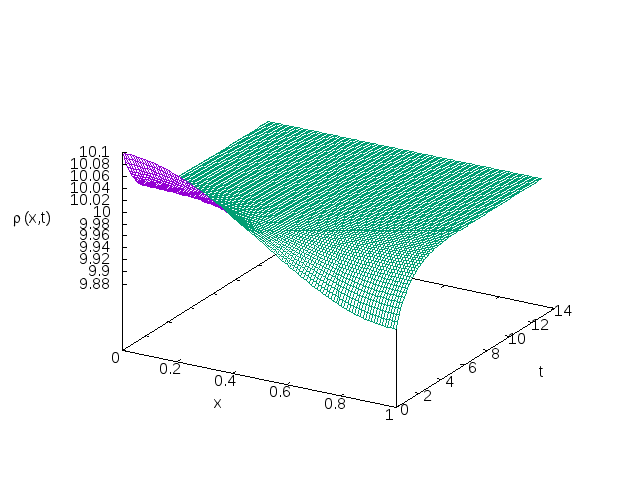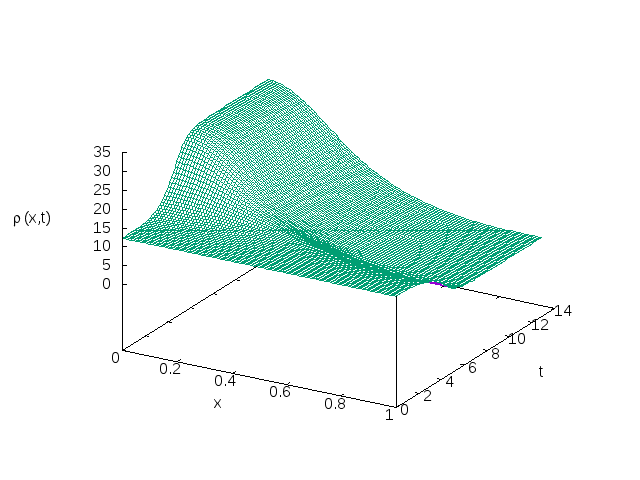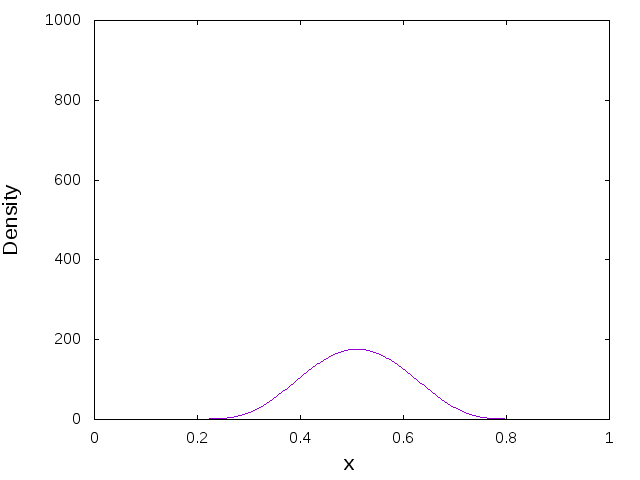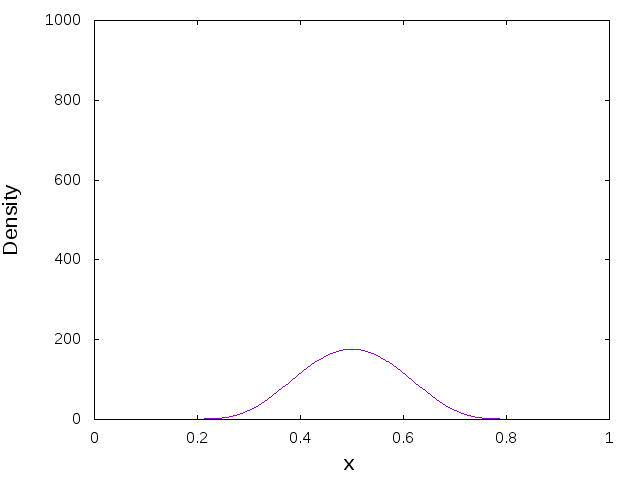# Numerical Simulations

#### Stability of Constant Solutions

For any$k \in \mathbb{R}_+$ the functions$\rho(x,t) \equiv c(x,t) \equiv k$ form a steady state solution. We investigated for a given perturbation whether the stability of the solution is dependent on$k$. The simulations seem to suggest this is indeed true. One example is given below. In this case$\Delta t=10^{-3}$,$h=5\times 10^{-3}$ and the initial condtions for the left hand and right hand figures are given by$\rho(x,0) = 10 + 0.1\text{cos}(\pi x)$ and$\rho(x,0) = 12 + 0.1\text{cos}(\pi x)$ respectively.#### Initial Data with One Peak

We consider a bump function$f_{x_0} : [0,1] \rightarrow \mathbb{R}$ defined by$f_{x_0}(x) := \begin{cases} 175 \exp \left(6.25-\frac{1}{0.16 - (x-x_0)^2}\right) & \quad \text{if } x \in (x_0 - 0.4, x_0 + 0.4) \\0& \quad \text{otherwise}\end{cases}$

Our simulations show that when$\rho(x,0) = 1 + f_{x_0}(x)$, the equations are very sensitive to the placement of$x_0$. When the peak is near the midpoint it drifts towards the closest boundary. For the two figures below the left hand one takes the value$x_0=0.49$, and the right hand one takes the value$x_0=0.51.$ For both simulations below we used$\Delta t=10^{-5}$,$h=2\times 10^{-3}$ and$T=0.15$.When the peak is located at the mid point,$\rho(x,t)$ appears to approach an equilibrium solution, but eventually the peak drifts off to the boundary. This behaviour seems very strange because the initial condition in this case is symmetric about the mid point and the equations suggest the evolution should be symmetric. We suspect that a combination of a machine rounding error and a translational instability is at fault here. An equilibrium solution with a peak at the mid point for a large mass in the parabolic-parabolic case was constructed asymptotically in . They also showed that this solution is unstable to translations, leading us to suspect a similar thing might be affecting our simulations. For the simulation below we used$\Delta t=10^{-4}$,$h=2 \times 10^{-3}$ and$T=1.5$,#### References

 K. Kang, T. Kolokolnikov and M. J. Ward, The stability and dynamics of a spike in the 1D Keller-Segel model, IMA journal of applied mathematics, 72 (2007), pp.140-162.